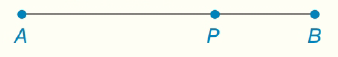Chapter 1.CT, Problem 3CT### Elementary Geometry for College St...

6th Edition
Daniel C. Alexander + 1 other
ISBN: 9781285195698

#### Solutions

Chapter
Section### Elementary Geometry for College St...

6th Edition
Daniel C. Alexander + 1 other
ISBN: 9781285195698
Textbook Problem
25 views

# Using the Segment-Addition Postulate, state a conclusion regarding the accompanying figure.To determine

To find:

The conclusion regarding the given figure.

Explanation

Given:

The given figure is,

Concept:

According to Segment-Addition Postulate, if two points A, B are given on a line segment, then third point C lies on the line segment if and only if,

AC+CB=AB

Approach:

From the given figure, it is clear that the points A, P and B are collinear and P lies between A and B

### Still sussing out bartleby?

Check out a sample textbook solution.

See a sample solution

#### The Solution to Your Study Problems

Bartleby provides explanations to thousands of textbook problems written by our experts, many with advanced degrees!

Get Started

#### Simplify: 918

Elementary Technical Mathematics

#### If g(x) = 3 + x + ex, find g1(4).

Single Variable Calculus: Early Transcendentals